Function Repository Resource:

# PermutationAscents

Give the indices of a permutation where there is an immediate ascent

Contributed by: Wolfram Staff
 ResourceFunction["PermutationAscents"][p] gives the indices i where pi

## Details and Options

List notation for a permutation is also called one-line notation.

## Examples

### Basic Examples (3)

Consider the permutation:

 In:=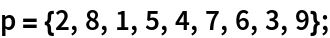Its ascents are at the indices 1, 3, 5, 8, corresponding to 2 < 8, 1 < 5, 4 < 7, 3 < 9:

 In:=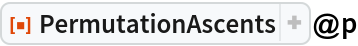Out=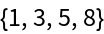In:=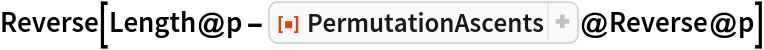Out=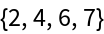### Possible Issues (4)

A permutation in cycle form:

 In:=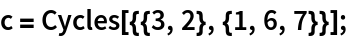This does not work:

 In:=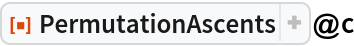Out=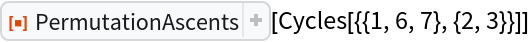Convert from cycle to list form:

 In:=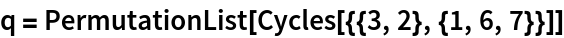Out=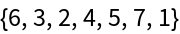Now this finds the ascents:

 In:=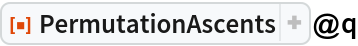Out=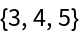George Beck

## Requirements

Wolfram Language 11.3 (March 2018) or above

## Version History

• 1.0.0 – 15 March 2019